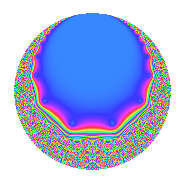# Properties

 Label 12.11.c.bLevel 12 Weight 11 Character orbit 12.c Analytic conductor 7.624 Analytic rank 0 Dimension 2 CM No Inner twists 2

# Related objects

## Newspace parameters

 Level: $$N$$ = $$12 = 2^{2} \cdot 3$$ Weight: $$k$$ = $$11$$ Character orbit: $$[\chi]$$ = 12.c (of order $$2$$ and degree $$1$$)

## Newform invariants

 Self dual: No Analytic conductor: $$7.62428703208$$ Analytic rank: $$0$$ Dimension: $$2$$ Coefficient field: $$\Q(\sqrt{-35})$$ Coefficient ring: $$\Z[a_1, a_2, a_3]$$ Coefficient ring index: $$2^{3}\cdot 3^{2}$$ Sato-Tate group: $\mathrm{SU}(2)[C_{2}]$

## $q$-expansion

Coefficients of the $$q$$-expansion are expressed in terms of $$\beta = 36\sqrt{-35}$$. We also show the integral $$q$$-expansion of the trace form.

 $$f(q)$$ $$=$$ $$q$$ $$+ ( 117 + \beta ) q^{3}$$ $$+ 6 \beta q^{5}$$ $$-10318 q^{7}$$ $$+ ( -31671 + 234 \beta ) q^{9}$$ $$+O(q^{10})$$ $$q$$ $$+ ( 117 + \beta ) q^{3}$$ $$+ 6 \beta q^{5}$$ $$-10318 q^{7}$$ $$+ ( -31671 + 234 \beta ) q^{9}$$ $$+ 1374 \beta q^{11}$$ $$-256822 q^{13}$$ $$+ ( -272160 + 702 \beta ) q^{15}$$ $$-2616 \beta q^{17}$$ $$+ 3196106 q^{19}$$ $$+ ( -1207206 - 10318 \beta ) q^{21}$$ $$-39228 \beta q^{23}$$ $$+ 8132665 q^{25}$$ $$+ ( -14319747 - 4293 \beta ) q^{27}$$ $$+ 147858 \beta q^{29}$$ $$+ 23140994 q^{31}$$ $$+ ( -62324640 + 160758 \beta ) q^{33}$$ $$-61908 \beta q^{35}$$ $$+ 29797946 q^{37}$$ $$+ ( -30048174 - 256822 \beta ) q^{39}$$ $$+ 4452 \beta q^{41}$$ $$+ 247522778 q^{43}$$ $$+ ( -63685440 - 190026 \beta ) q^{45}$$ $$-1547352 \beta q^{47}$$ $$-176014125 q^{49}$$ $$+ ( 118661760 - 306072 \beta ) q^{51}$$ $$+ 2588478 \beta q^{53}$$ $$-373947840 q^{55}$$ $$+ ( 373944402 + 3196106 \beta ) q^{57}$$ $$+ 1686054 \beta q^{59}$$ $$-1054839766 q^{61}$$ $$+ ( 326781378 - 2414412 \beta ) q^{63}$$ $$-1540932 \beta q^{65}$$ $$-361186198 q^{67}$$ $$+ ( 1779382080 - 4589676 \beta ) q^{69}$$ $$-4373844 \beta q^{71}$$ $$+ 374437394 q^{73}$$ $$+ ( 951521805 + 8132665 \beta ) q^{75}$$ $$-14176932 \beta q^{77}$$ $$-113914462 q^{79}$$ $$+ ( -1480679919 - 14822028 \beta ) q^{81}$$ $$+ 23058234 \beta q^{83}$$ $$+ 711970560 q^{85}$$ $$+ ( -6706838880 + 17299386 \beta ) q^{87}$$ $$+ 15410172 \beta q^{89}$$ $$+ 2649889396 q^{91}$$ $$+ ( 2707496298 + 23140994 \beta ) q^{93}$$ $$+ 19176636 \beta q^{95}$$ $$+ 2809917122 q^{97}$$ $$+ ( -14583965760 - 43515954 \beta ) q^{99}$$ $$+O(q^{100})$$ $$\operatorname{Tr}(f)(q)$$ $$=$$ $$2q$$ $$\mathstrut +\mathstrut 234q^{3}$$ $$\mathstrut -\mathstrut 20636q^{7}$$ $$\mathstrut -\mathstrut 63342q^{9}$$ $$\mathstrut +\mathstrut O(q^{10})$$ $$2q$$ $$\mathstrut +\mathstrut 234q^{3}$$ $$\mathstrut -\mathstrut 20636q^{7}$$ $$\mathstrut -\mathstrut 63342q^{9}$$ $$\mathstrut -\mathstrut 513644q^{13}$$ $$\mathstrut -\mathstrut 544320q^{15}$$ $$\mathstrut +\mathstrut 6392212q^{19}$$ $$\mathstrut -\mathstrut 2414412q^{21}$$ $$\mathstrut +\mathstrut 16265330q^{25}$$ $$\mathstrut -\mathstrut 28639494q^{27}$$ $$\mathstrut +\mathstrut 46281988q^{31}$$ $$\mathstrut -\mathstrut 124649280q^{33}$$ $$\mathstrut +\mathstrut 59595892q^{37}$$ $$\mathstrut -\mathstrut 60096348q^{39}$$ $$\mathstrut +\mathstrut 495045556q^{43}$$ $$\mathstrut -\mathstrut 127370880q^{45}$$ $$\mathstrut -\mathstrut 352028250q^{49}$$ $$\mathstrut +\mathstrut 237323520q^{51}$$ $$\mathstrut -\mathstrut 747895680q^{55}$$ $$\mathstrut +\mathstrut 747888804q^{57}$$ $$\mathstrut -\mathstrut 2109679532q^{61}$$ $$\mathstrut +\mathstrut 653562756q^{63}$$ $$\mathstrut -\mathstrut 722372396q^{67}$$ $$\mathstrut +\mathstrut 3558764160q^{69}$$ $$\mathstrut +\mathstrut 748874788q^{73}$$ $$\mathstrut +\mathstrut 1903043610q^{75}$$ $$\mathstrut -\mathstrut 227828924q^{79}$$ $$\mathstrut -\mathstrut 2961359838q^{81}$$ $$\mathstrut +\mathstrut 1423941120q^{85}$$ $$\mathstrut -\mathstrut 13413677760q^{87}$$ $$\mathstrut +\mathstrut 5299778792q^{91}$$ $$\mathstrut +\mathstrut 5414992596q^{93}$$ $$\mathstrut +\mathstrut 5619834244q^{97}$$ $$\mathstrut -\mathstrut 29167931520q^{99}$$ $$\mathstrut +\mathstrut O(q^{100})$$

## Character Values

We give the values of $$\chi$$ on generators for $$\left(\mathbb{Z}/12\mathbb{Z}\right)^\times$$.

 $$n$$ $$5$$ $$7$$ $$\chi(n)$$ $$-1$$ $$1$$

## Embeddings

For each embedding $$\iota_m$$ of the coefficient field, the values $$\iota_m(a_n)$$ are shown below.

For more information on an embedded modular form you can click on its label.

Label $$\iota_m(\nu)$$ $$a_{2}$$ $$a_{3}$$ $$a_{4}$$ $$a_{5}$$ $$a_{6}$$ $$a_{7}$$ $$a_{8}$$ $$a_{9}$$ $$a_{10}$$
5.1
 0.5 − 2.95804i 0.5 + 2.95804i
0 117.000 212.979i 0 1277.87i 0 −10318.0 0 −31671.0 49837.1i 0
5.2 0 117.000 + 212.979i 0 1277.87i 0 −10318.0 0 −31671.0 + 49837.1i 0
 $$n$$: e.g. 2-40 or 990-1000 Significant digits: Format: Complex embeddings Normalized embeddings Satake parameters Satake angles

## Inner twists

Char. orbit Parity Mult. Self Twist Proved
1.a Even 1 trivial yes
3.b Odd 1 yes

## Hecke kernels

This newform can be constructed as the kernel of the linear operator $$T_{5}^{2}$$ $$\mathstrut +\mathstrut 1632960$$ acting on $$S_{11}^{\mathrm{new}}(12, [\chi])$$.